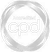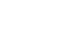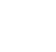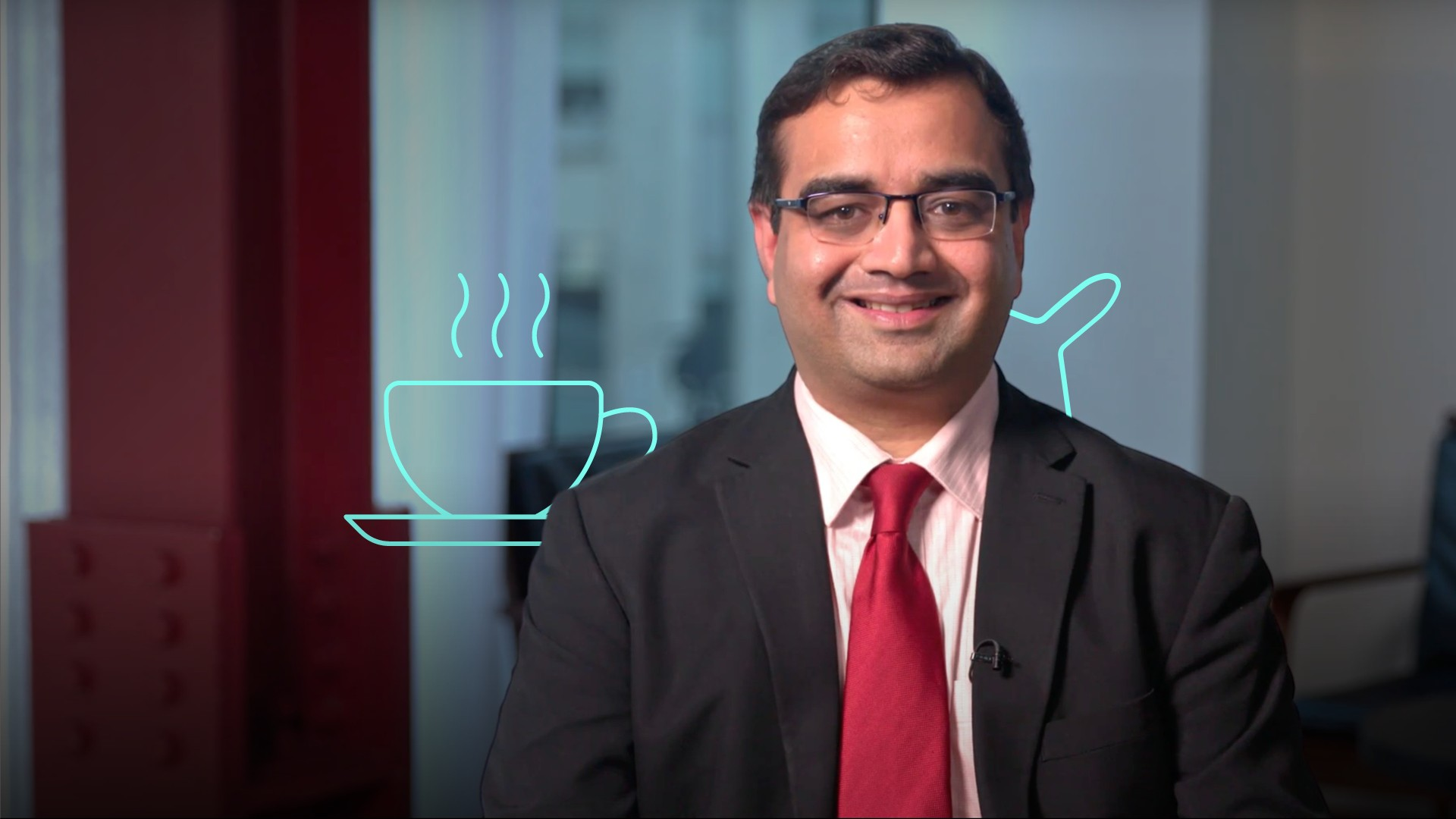# IFRS 16 Lessee Presentation### Saket Modi

20 years: Chartered accountant & educator

In this video, Saket outlines the impact of IFRS 16 on the balance sheet. He provides two examples; one explaining the application of the definition of lease in IFRS 16, the other demonstrates a situation where the contract fails to meet the definition of a lease.

Accounting FundamentalsIn this video, Saket outlines the impact of IFRS 16 on the balance sheet. He provides two examples; one explaining the application of the definition of lease in IFRS 16, the other demonstrates a situation where the contract fails to meet the definition of a lease.

Finance Unlocked is the video learning platform built for finance professionals.### IFRS 16 Lessee Presentation

9 mins 57 secs

Overview

In this video, Saket outlines the impact of IFRS 16 on the balance sheet, and how to determine the initial recognition and the subsequent measurement of lease liability and of the right-of-use asset.

Key learning objectives:

• Understand how to determine the initial recognition and the subsequent measurement of lease liability

• Understand how to determine the initial recognition and the subsequent measurement of the right-of-use asset

• Outline the profit or loss impact of the lease

## Book a demo to access

Summary

#### What is the impact of IFRS 16 on the balance sheet?

Air France-KLM Group has adopted IFRS 16 Leases and disclosed at 31 of December 2018.

• Right-of-use assets of €5.2bn
• Lease debt of €4.5bn (non-current liability of €3.5bn and currently liability of €1bn)
• There is a difference of €0.7bn between the right-of-use asset and the lease liability recognised. This is because the amortisation of principal amount of the lease liability is on a different basis to the depreciation of the right-of-use asset

#### Using the information below, how do we determine the (a) initial recognition and the subsequent measurement of (b) lease liability?

An entity leases a car on the following terms:

• Term: Three years
• Annual payment in arrears: £7200
• Discount rate: 3.95% - the lessee's incremental borrowing rate
• The annual payment of £7200 includes up to 25,000 miles. Each additional mile is charged at £0.20.

The entity used the car as follows:

• Year 1: 20,000 miles
• Year 2: 25,000 miles
• Year 3: 30,000 miles

(a) The lease liability is initially recognised at the present value of these lease payments. The present value is shown in the table below: The lease liability of £20,000 is the sum of the present value of each of the three lease payments of £7,200 discounted at the rate of 3.95%.(b) The subsequent measure of lease liability is as follows: Interest = 20,000 x 3.95% = 790. End of year lease liability = 20,000 - (7,200 - 790) = £13,590.#### What are the key points in the above example?

• The finance cost is higher in the early years of the lease due to higher liability balance
• The lease payment comprises of interest and principal components
• The interest component can be presented as cash outflow from operating or financing activities
• The end of year lease liability is split between non-current and current liability

#### Using the same information, how do we determine the (a) initial recognition and the subsequent measurement of the (b) right-of-use asset?

In addition to the above, the initial direct costs of entering into the lease are £1,000.

(a) Using the present value table from above, the right-of-use asset is initially recognised at £20,000 + £1,000 = £21,000. This includes initial direct costs of £1,000.

(b) The subsequent measurement of the right-of-use asset is shown in the table below: The right-of-use asset is depreciated on a straight-line basis over the lease term since the asset would be returned back to the lessor at the end of the three years.#### What is the profit or loss impact of the lease?#### What are the key points from the above example?

• The finance cost is calculated at the original discount rate of 3.95% on the lease liability at the beginning of the year
• The total expense consists of depreciation and finance cost
• Since the total expense is higher in the early years of the lease, the net income is relatively lower compared to the later years of the lease
• The right-of-use asset and lease liability is presented either as separately on face of the statement of financial position, or included in the same line item as similar assets/liabilities and disclosed separately in the notes

## Book a demo to access

Expert### Saket Modi

Saket is a financial trainer and consultant based out of London. He specialises in advanced accounting, financial reporting and financial analysis, particularly with regards to International Financial Reporting Standards (IFRS), International Public Sector Accounting Standards (IPSAS) and Financial instruments.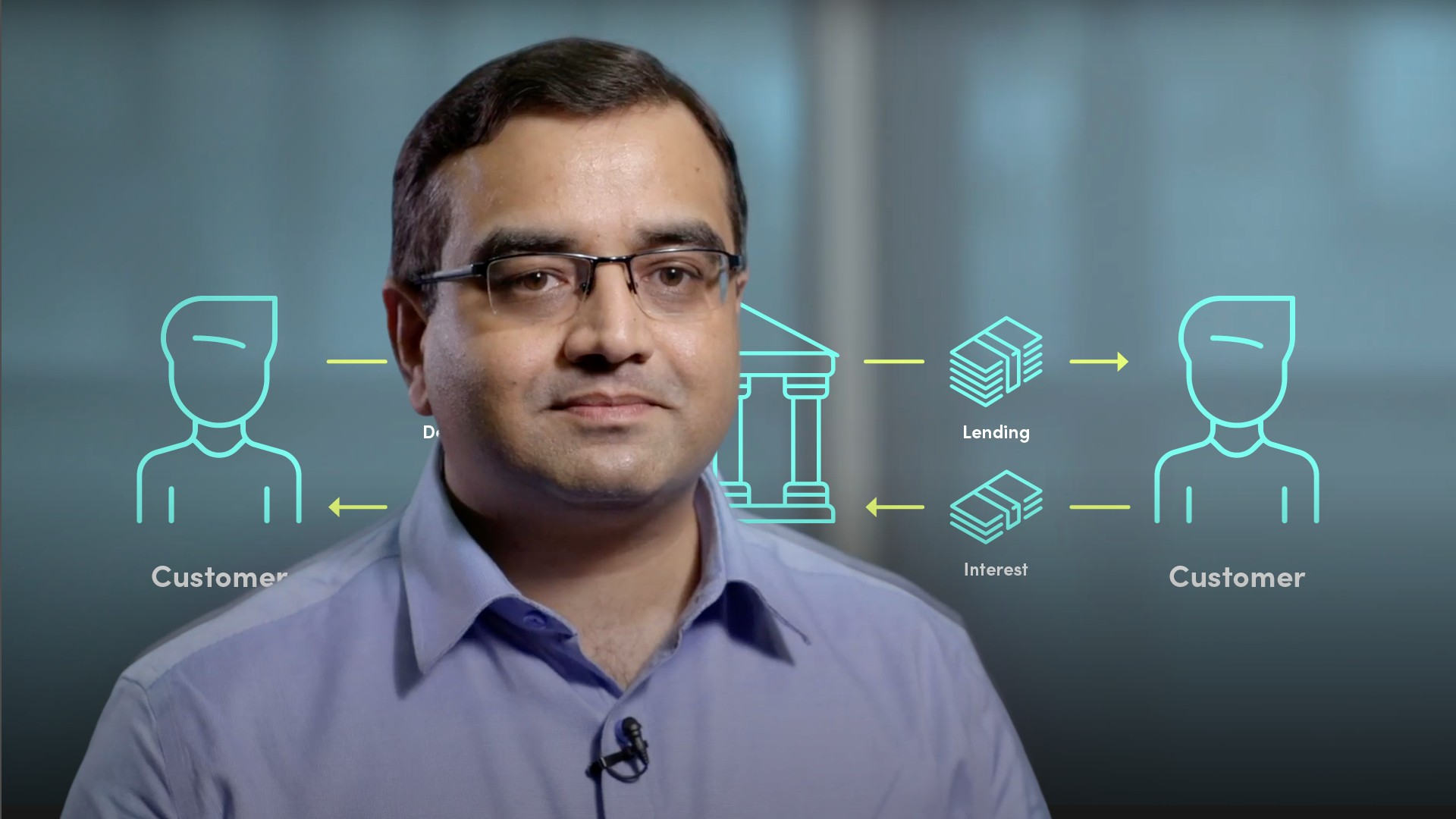06:24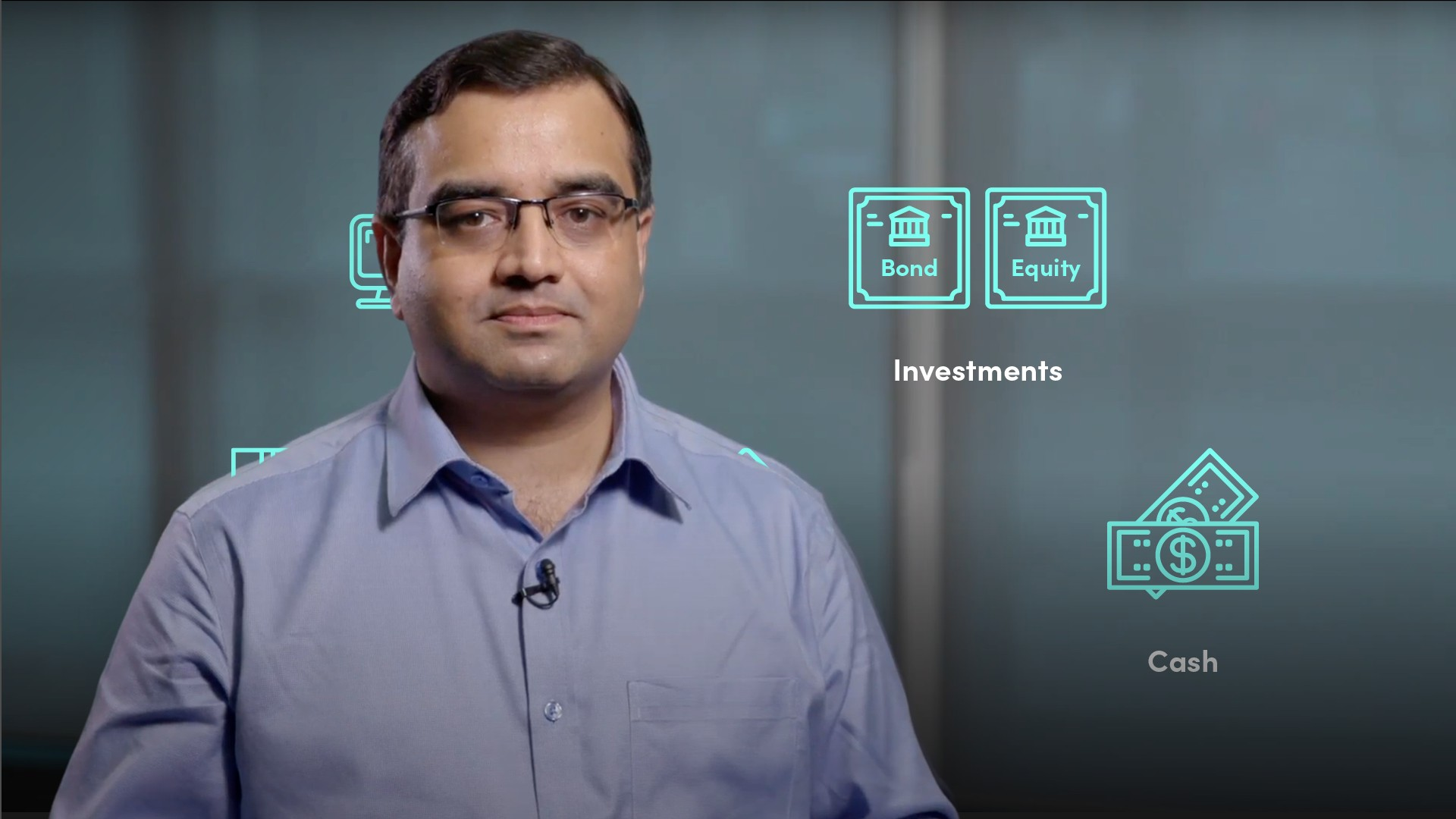08:52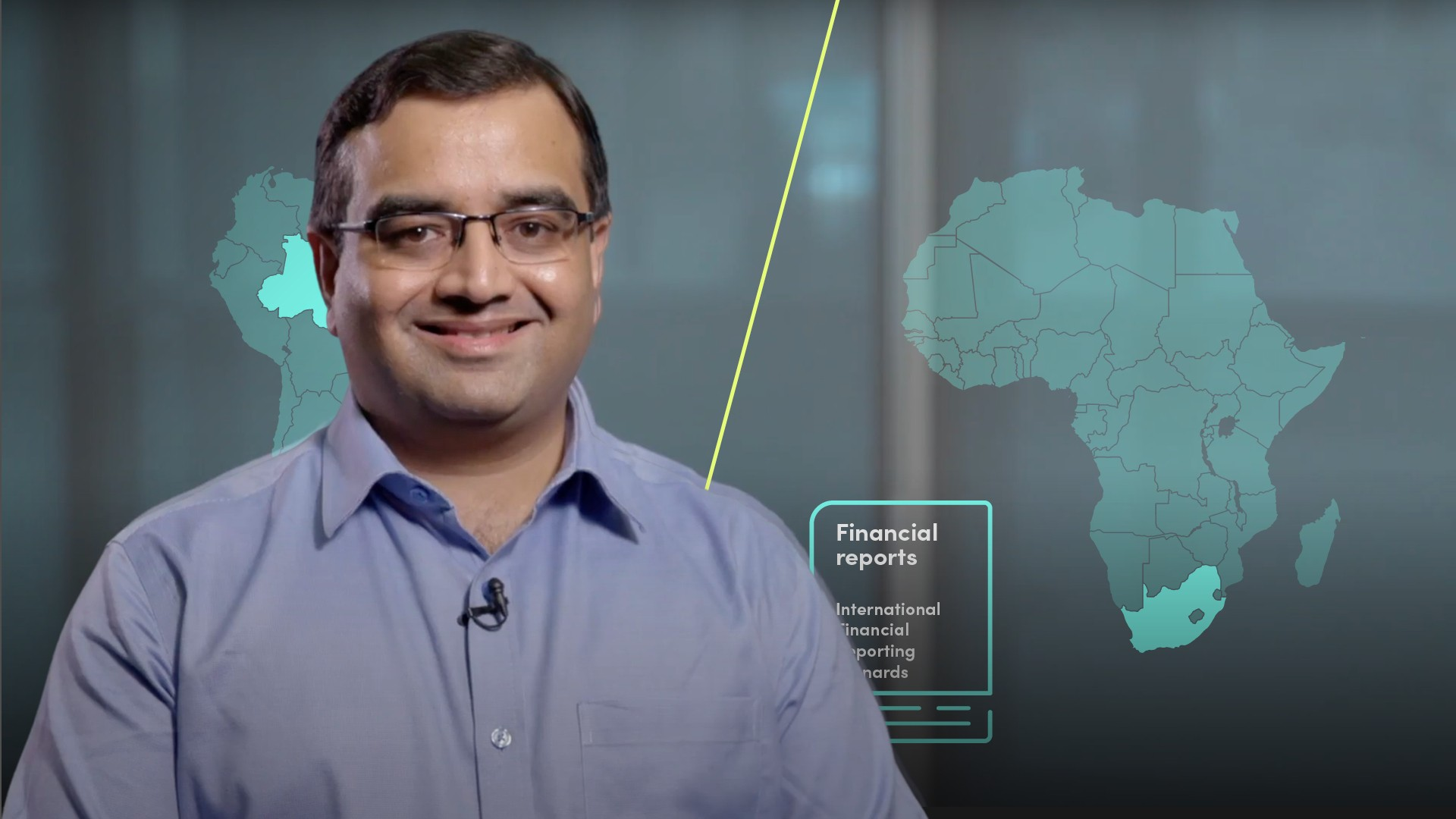05:2903:35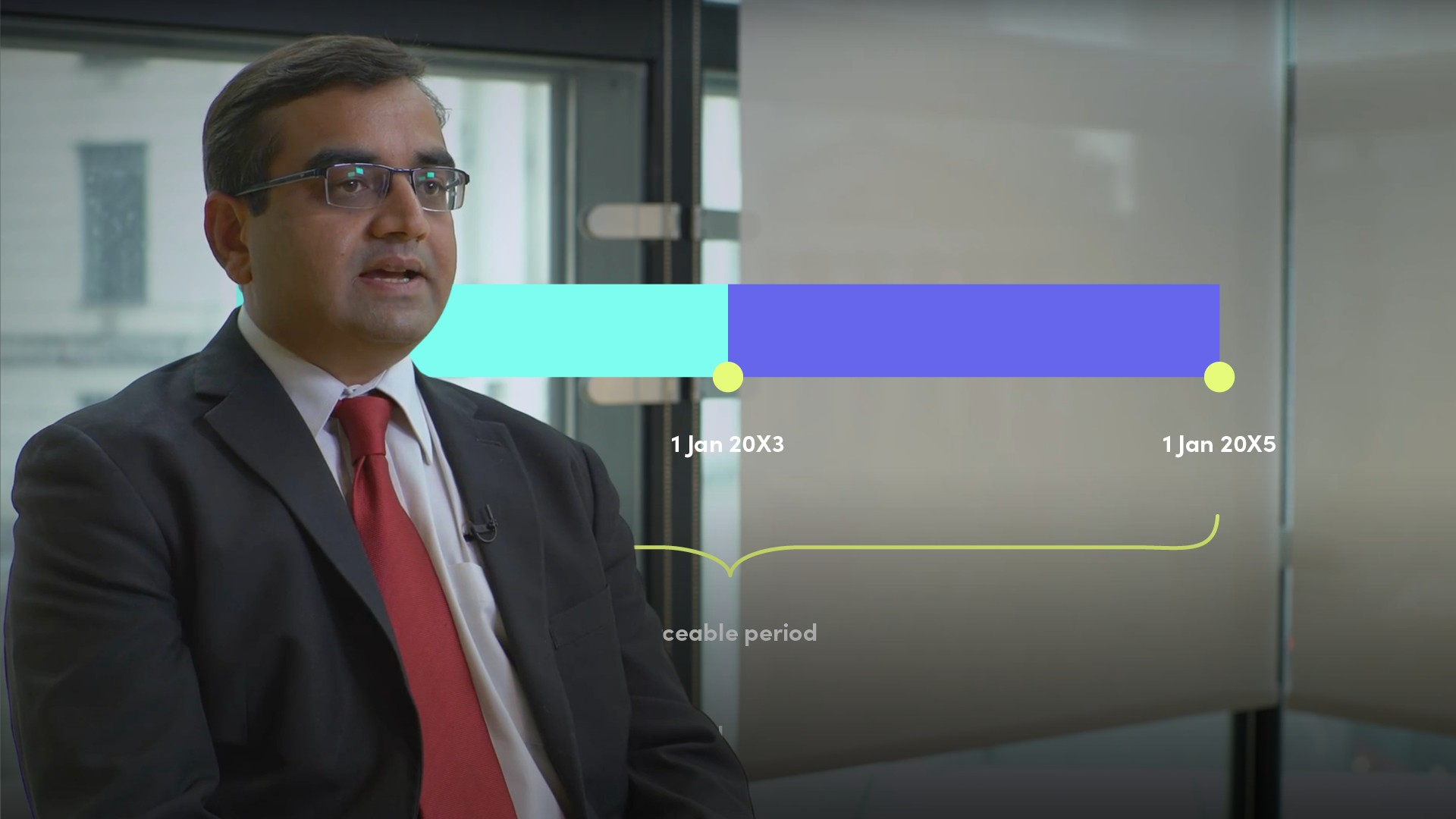01:04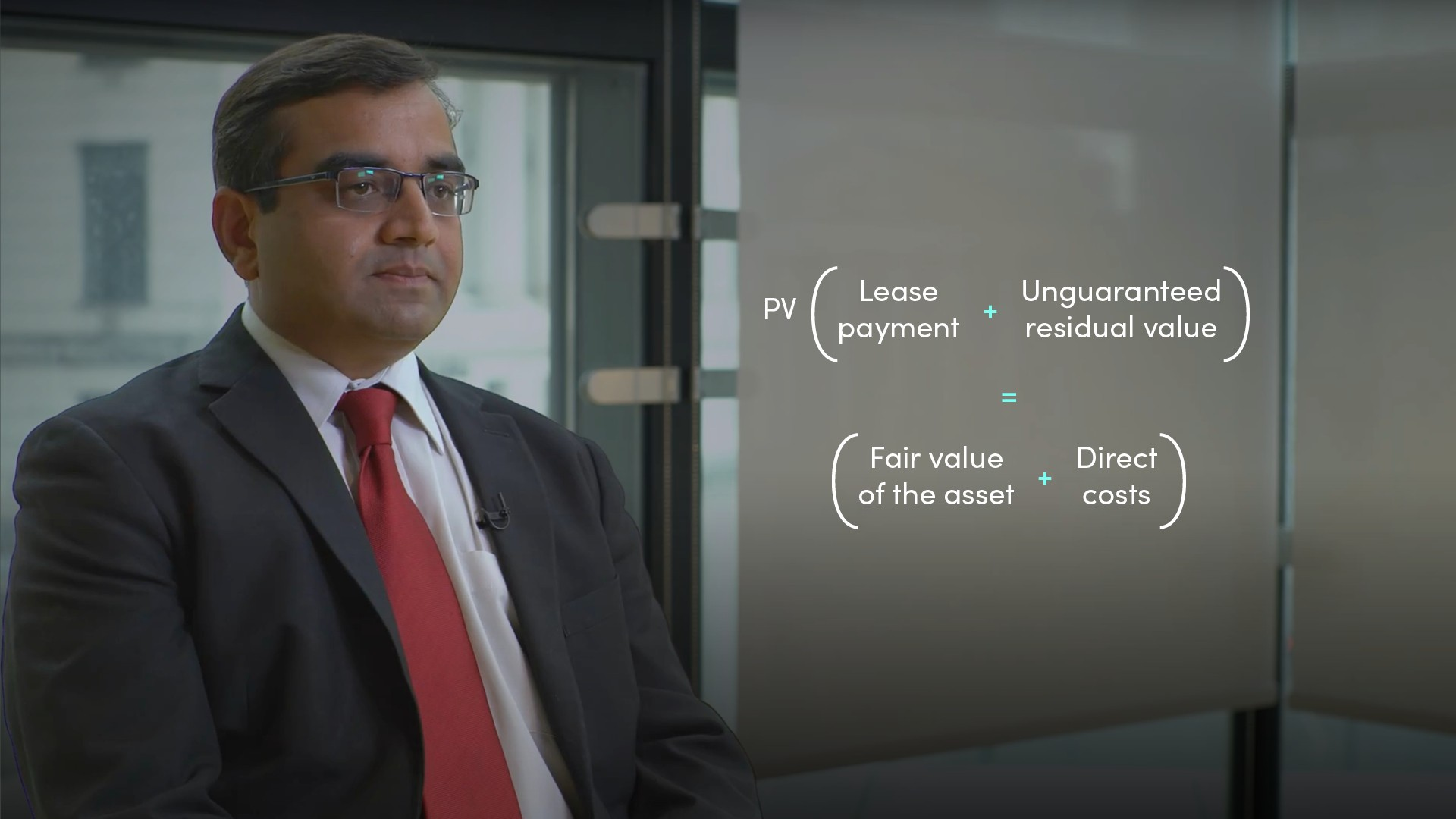01:04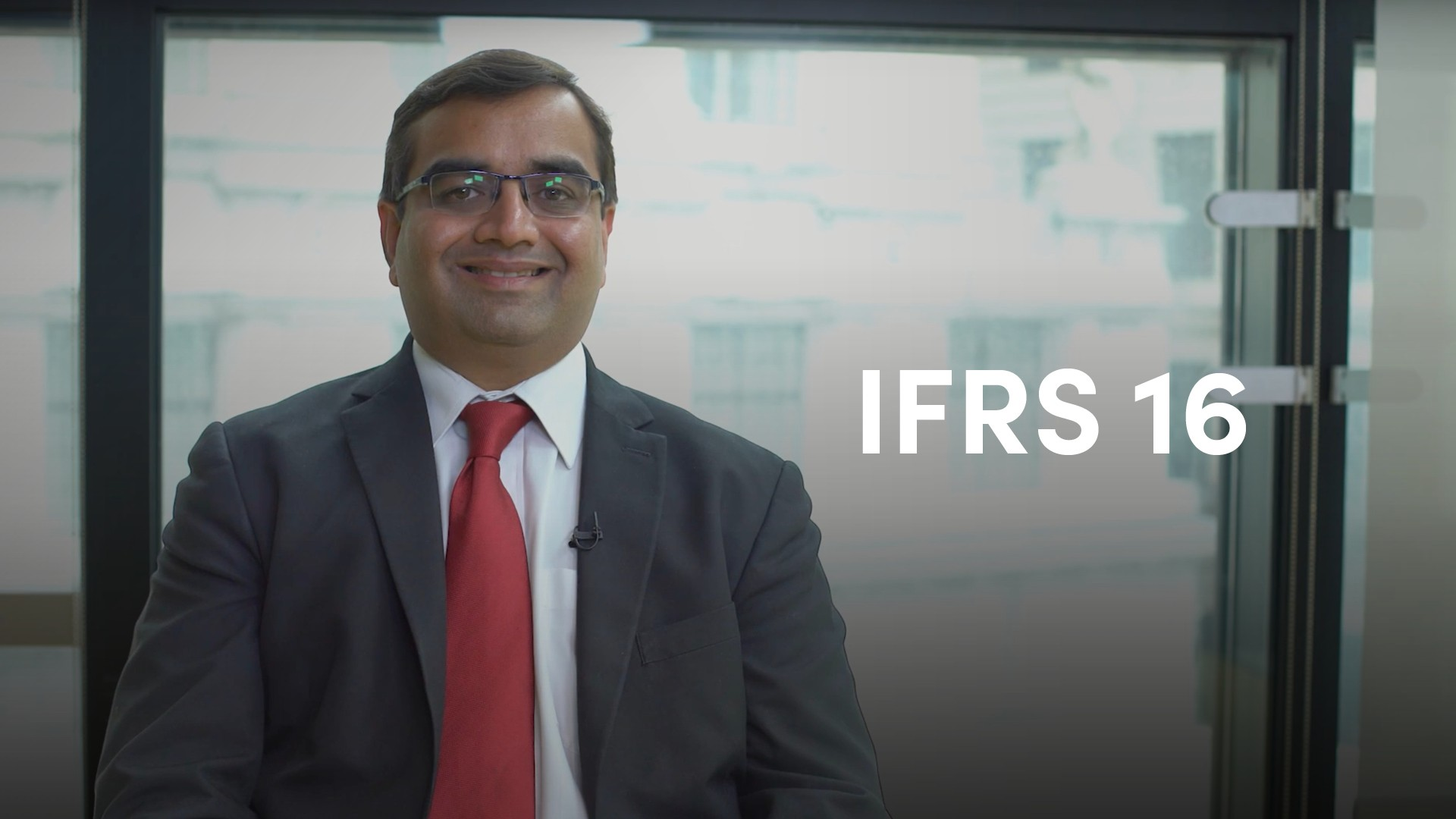02:5103:56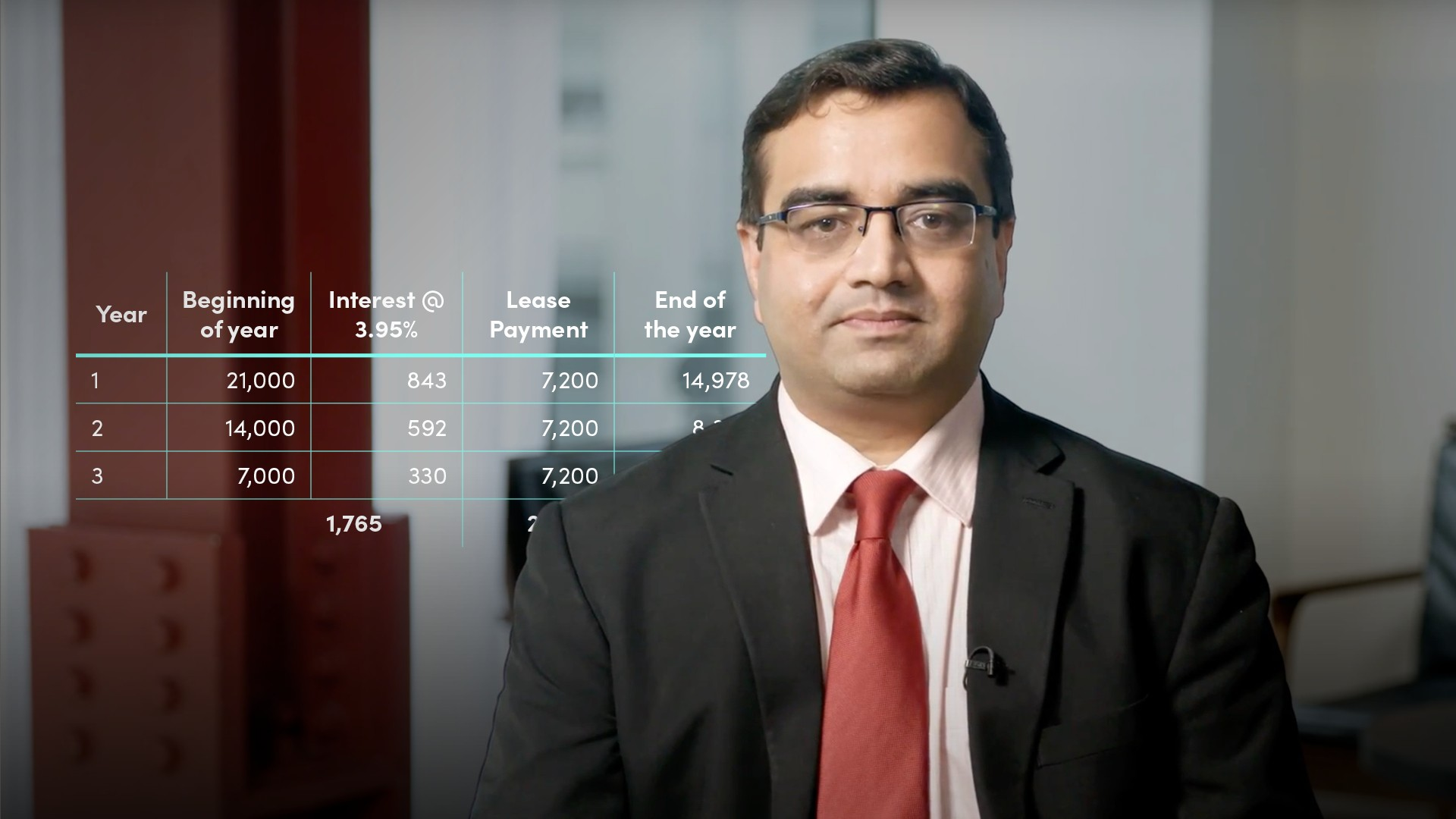04:51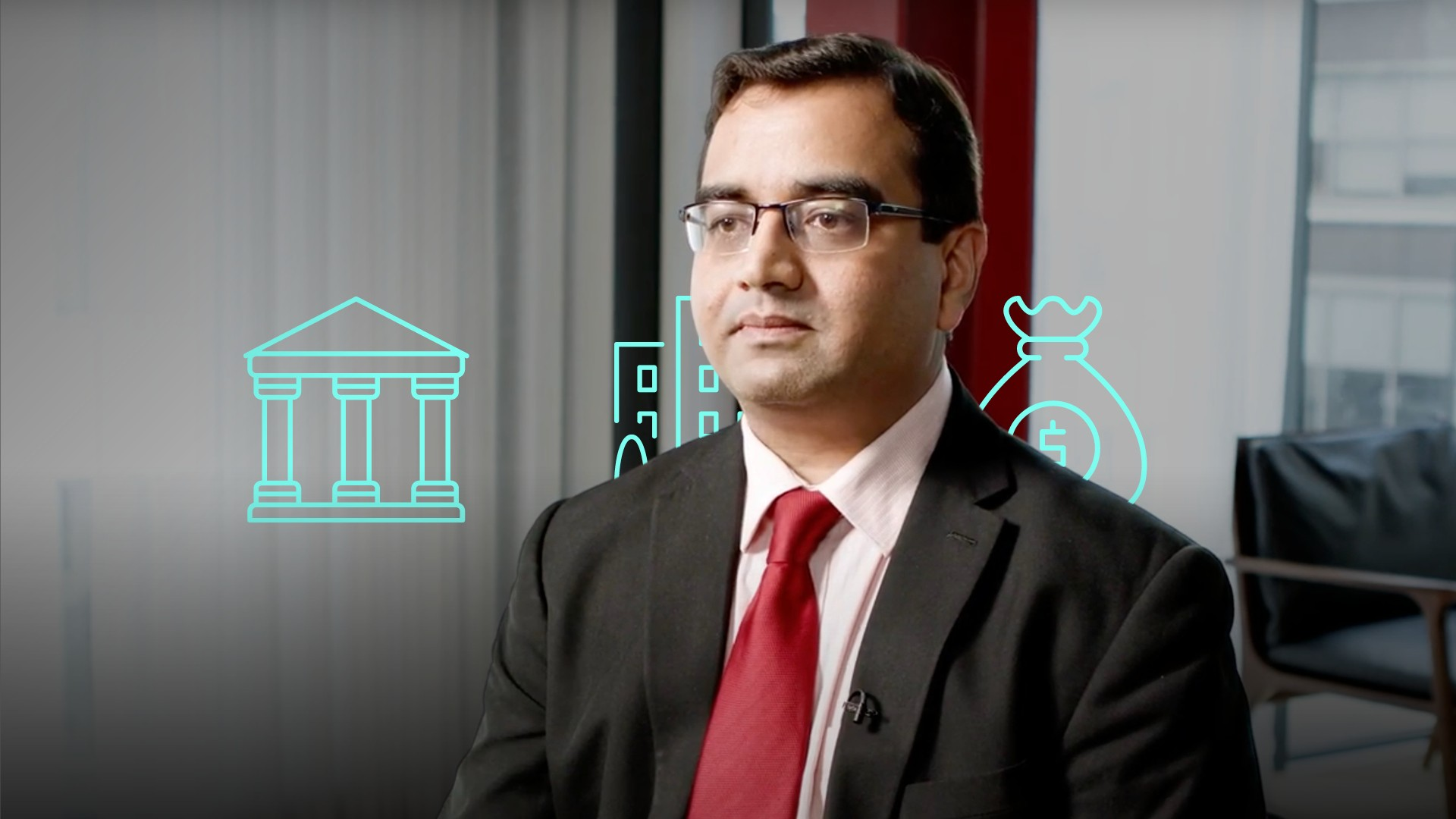05:28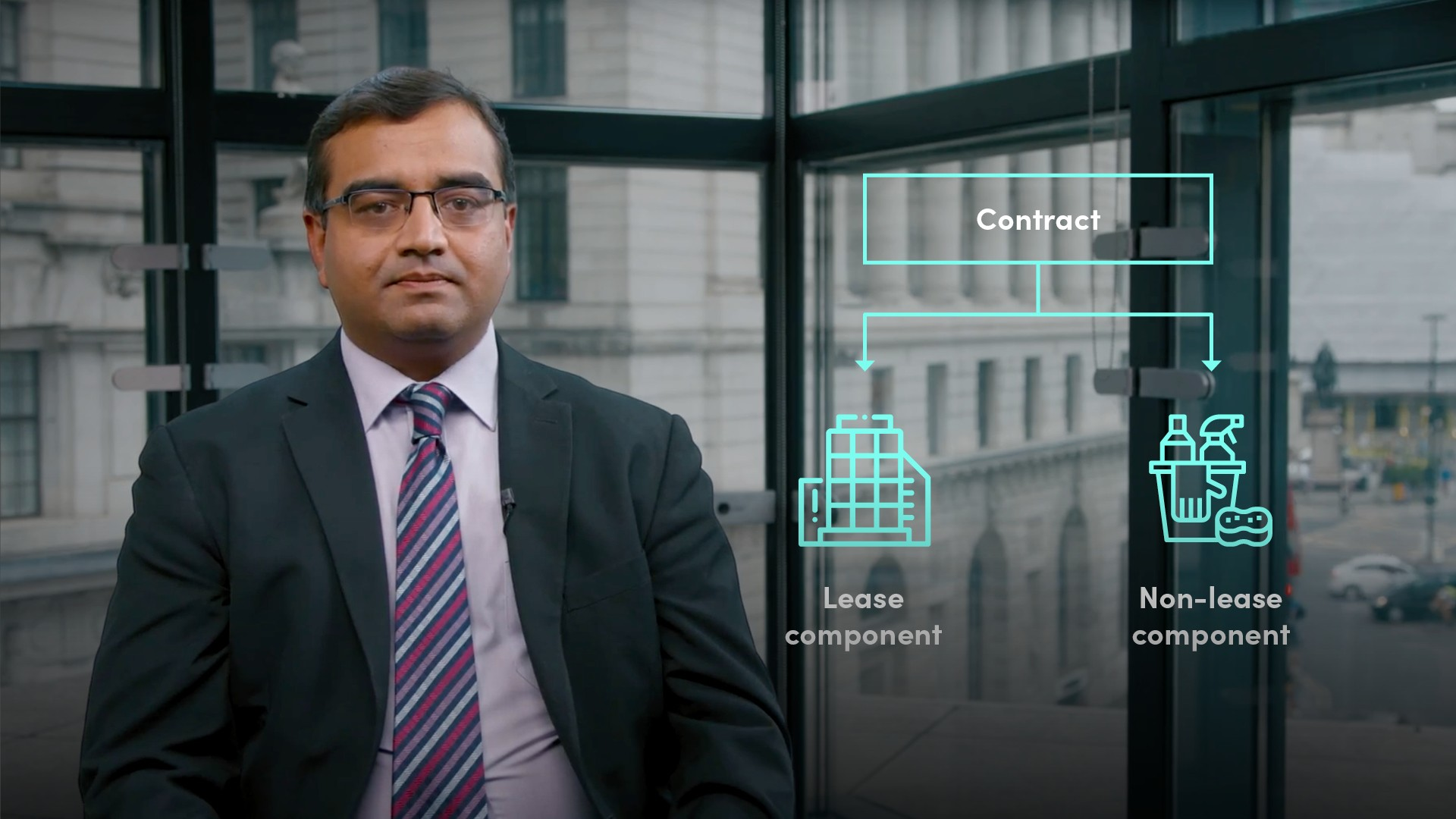08:55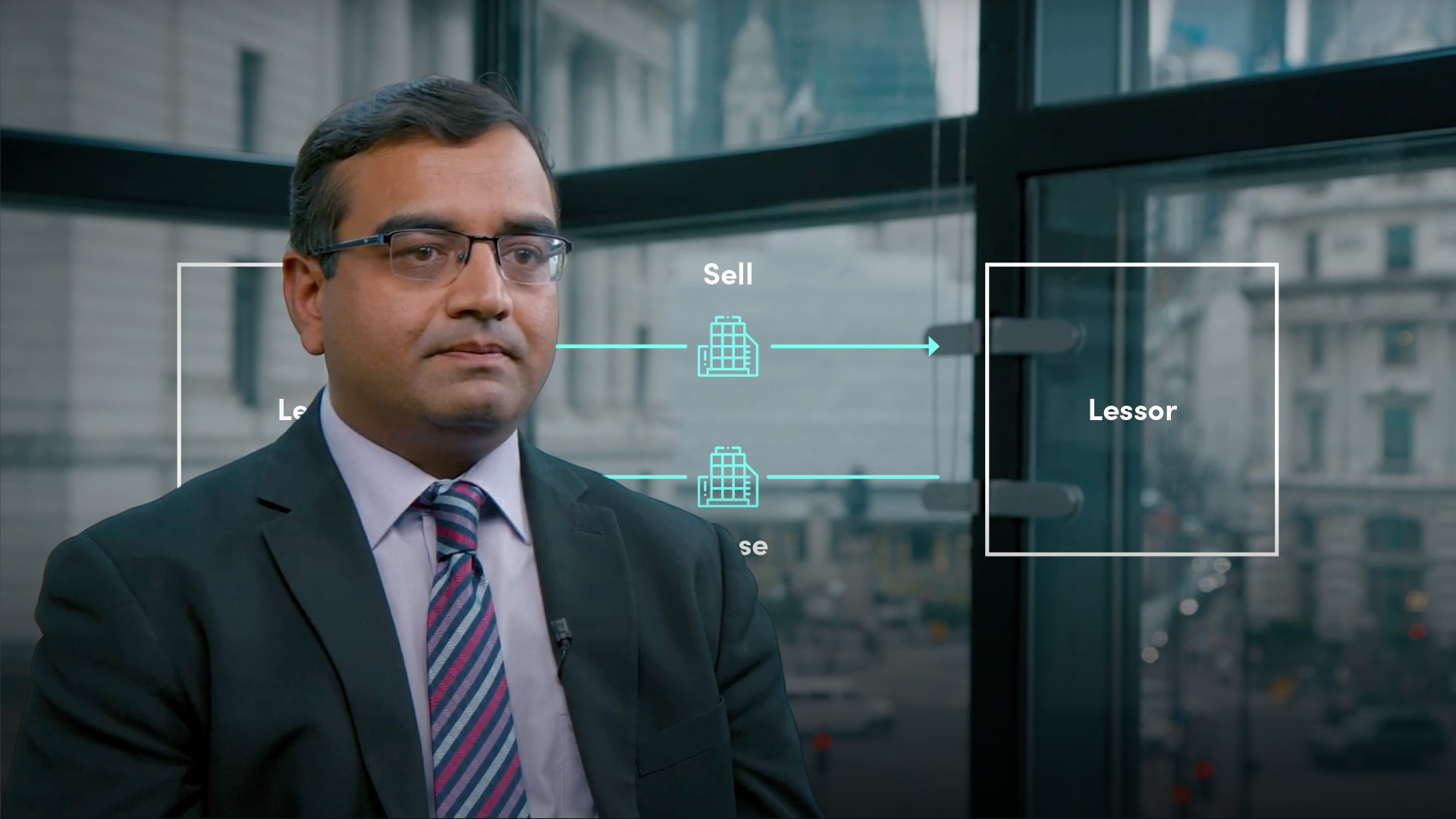06:2417:19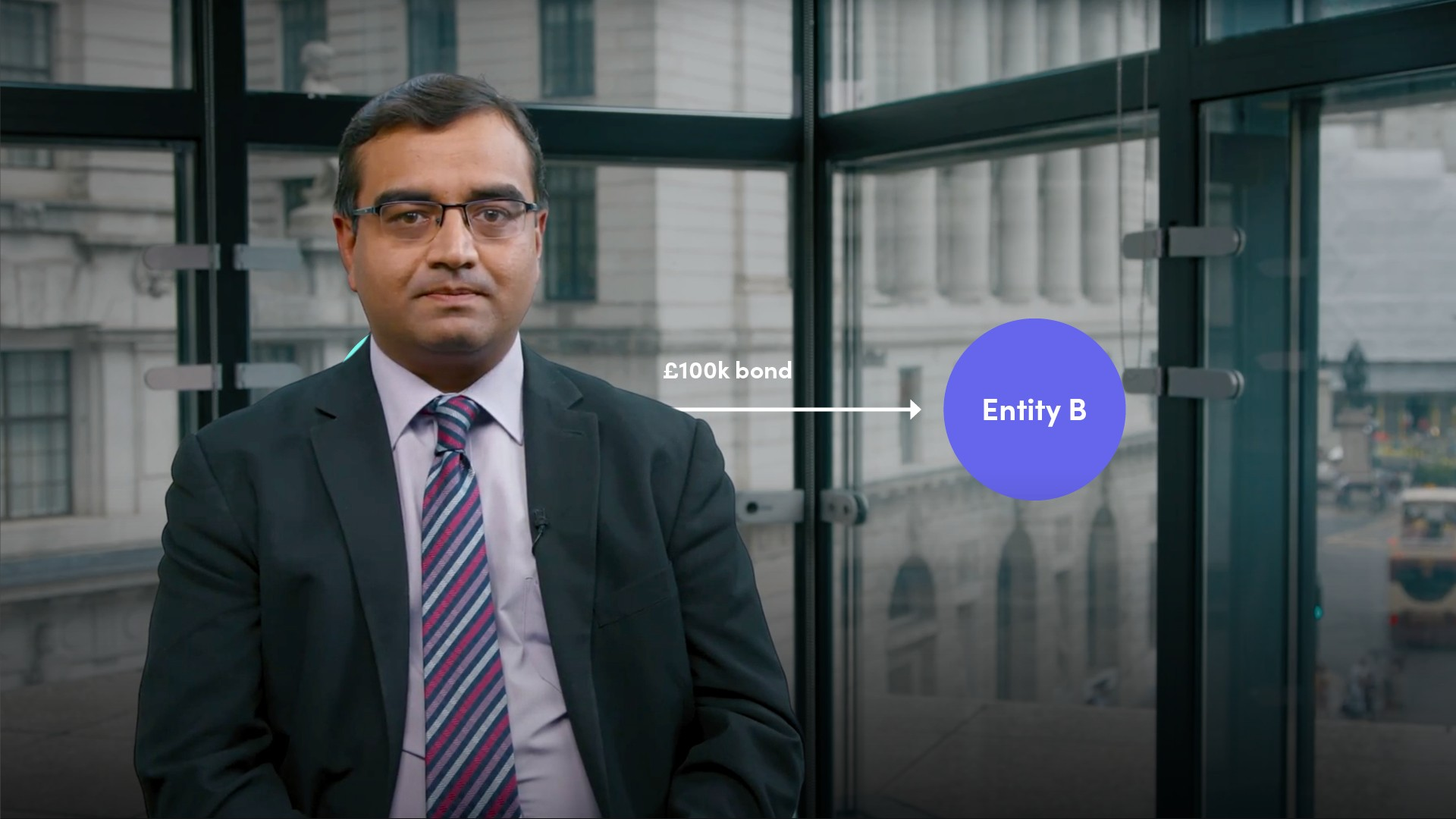12:19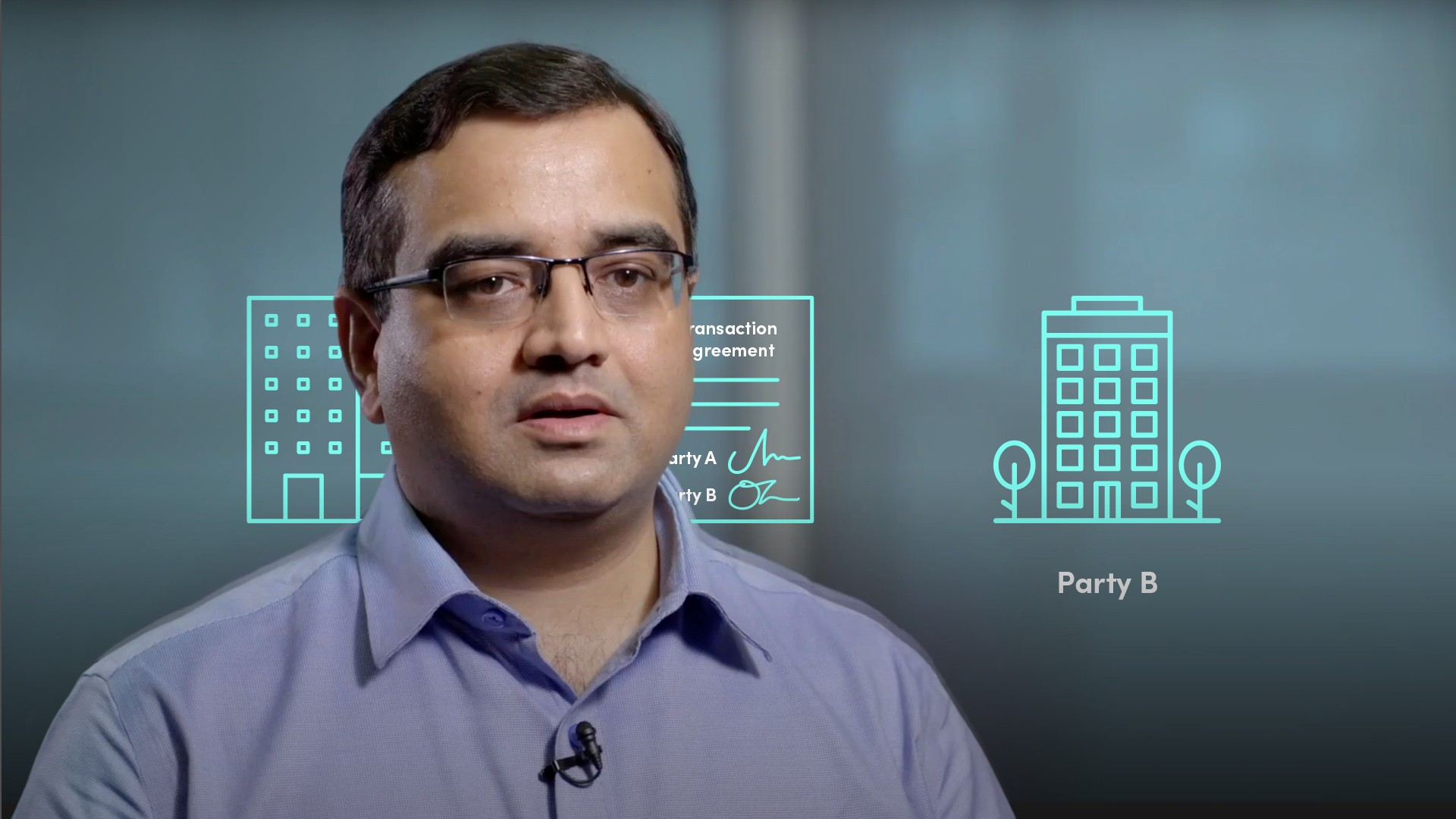03:13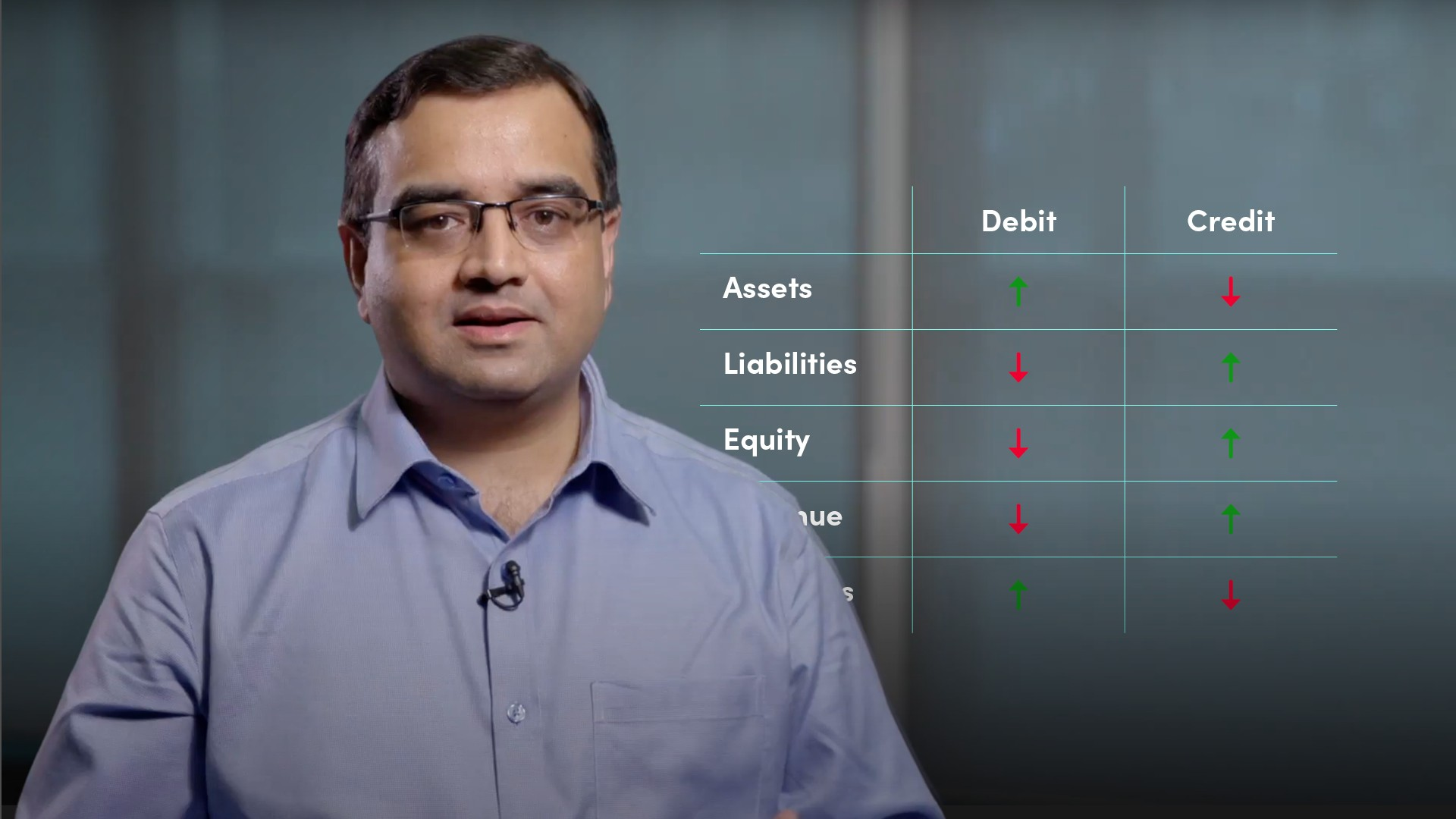05:16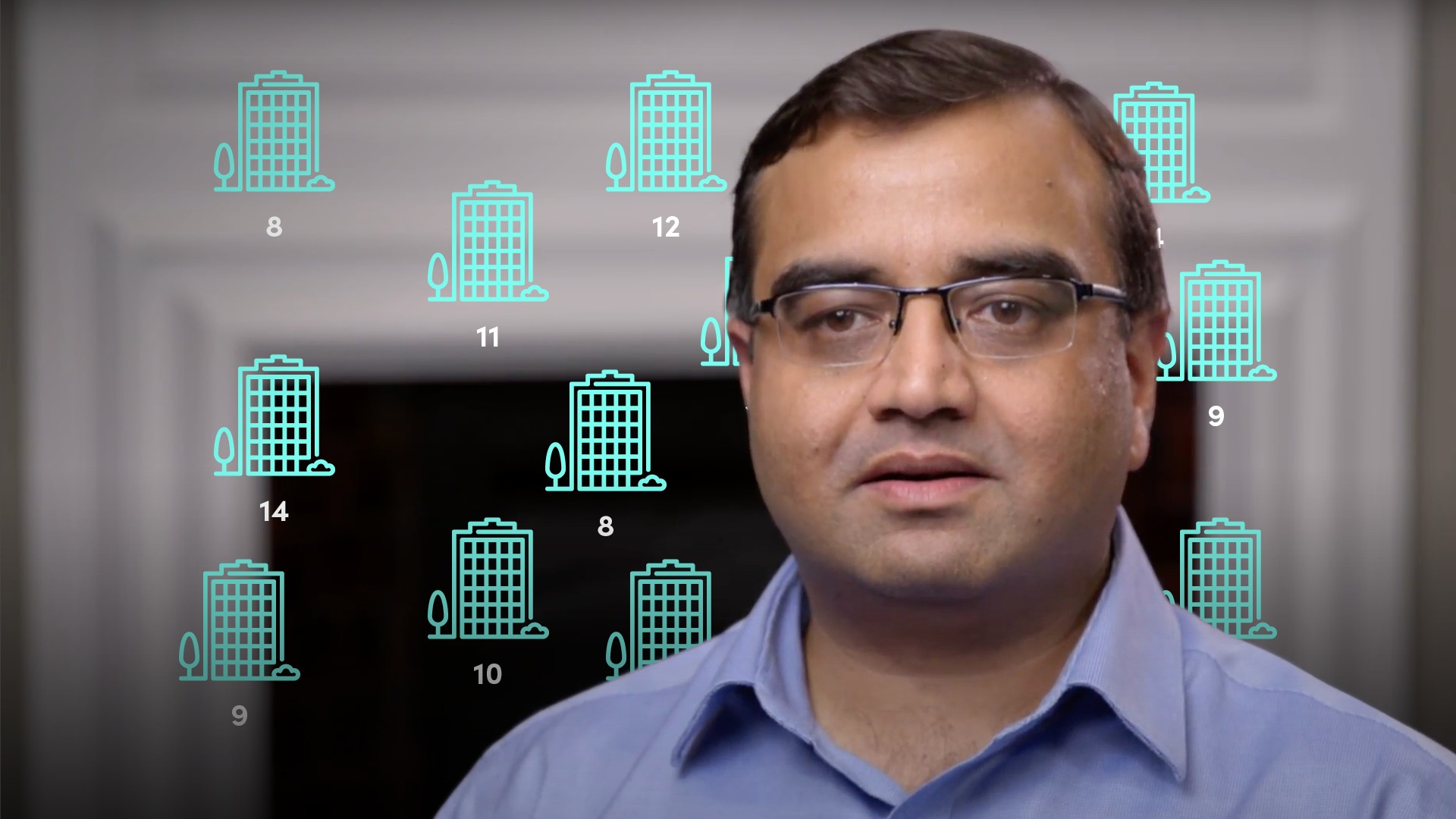04:58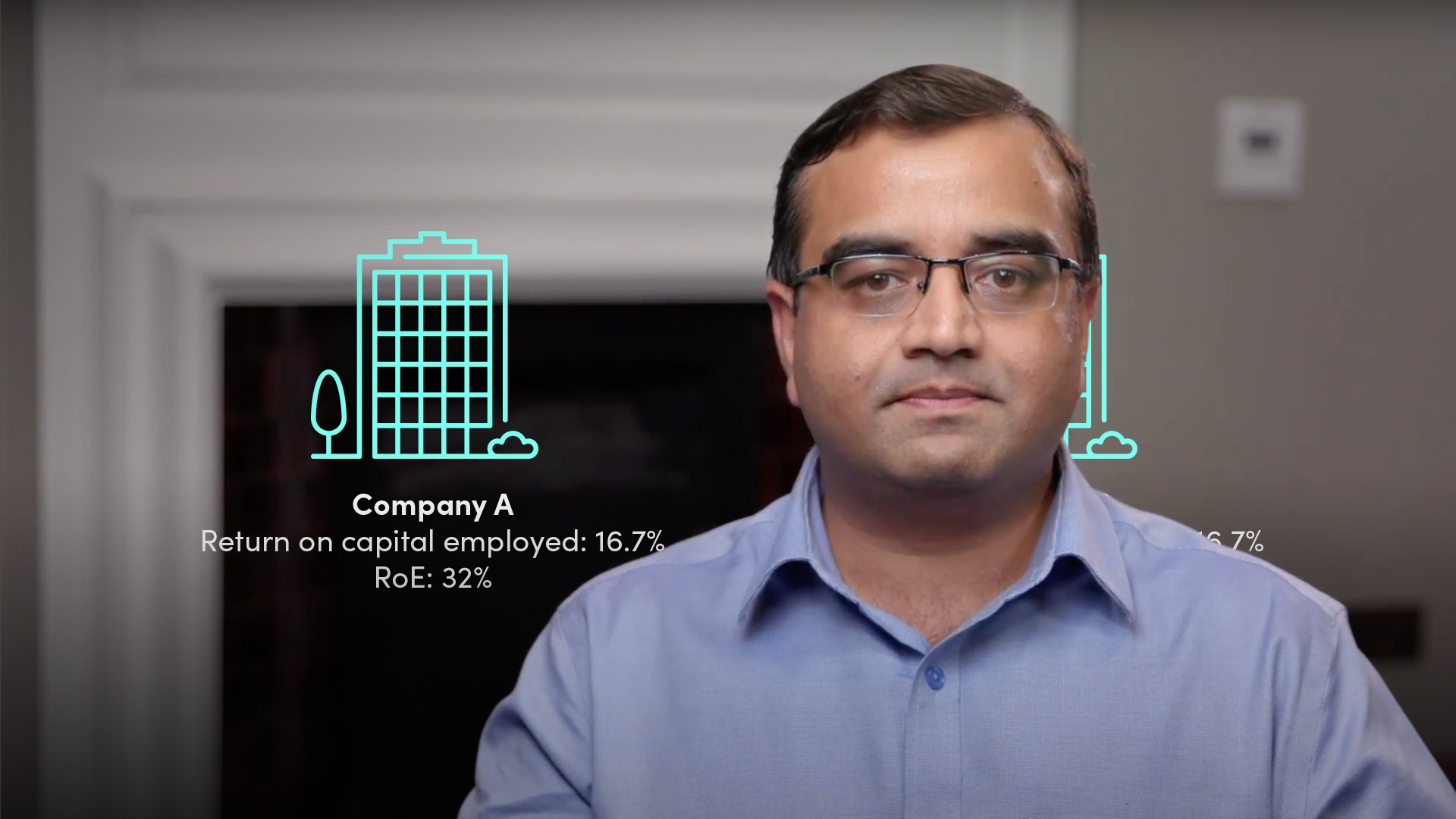04:16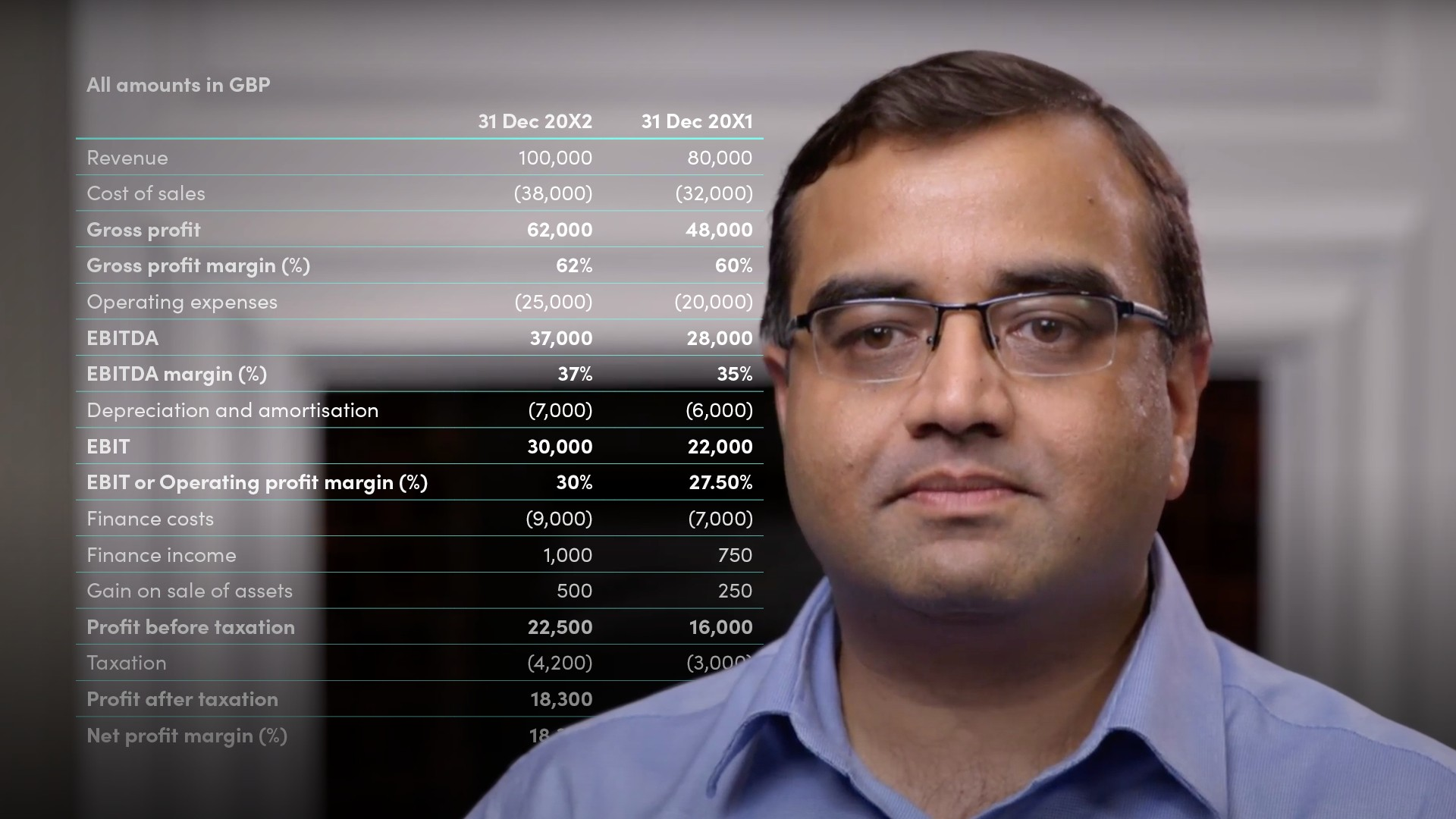06:20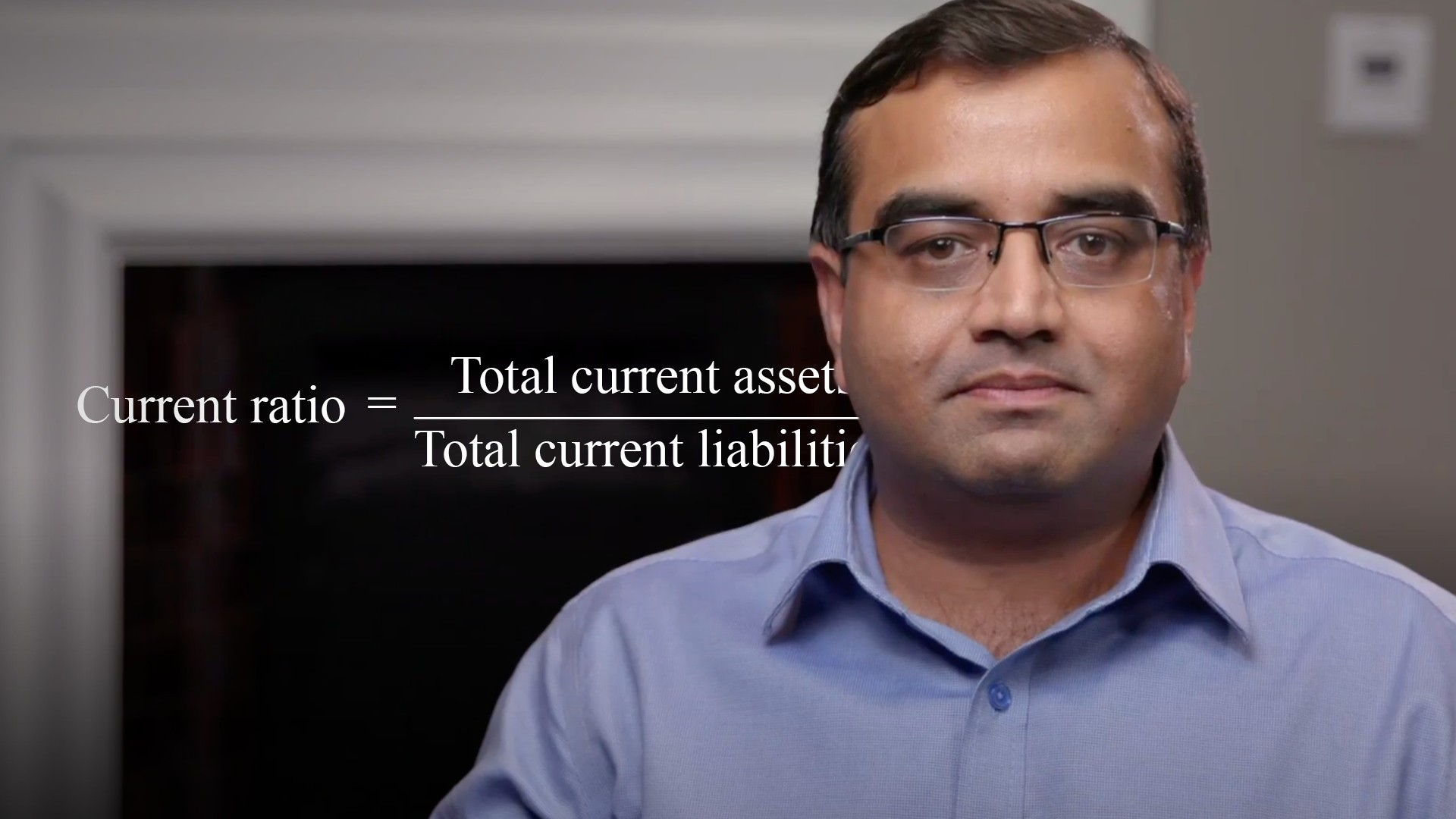03:34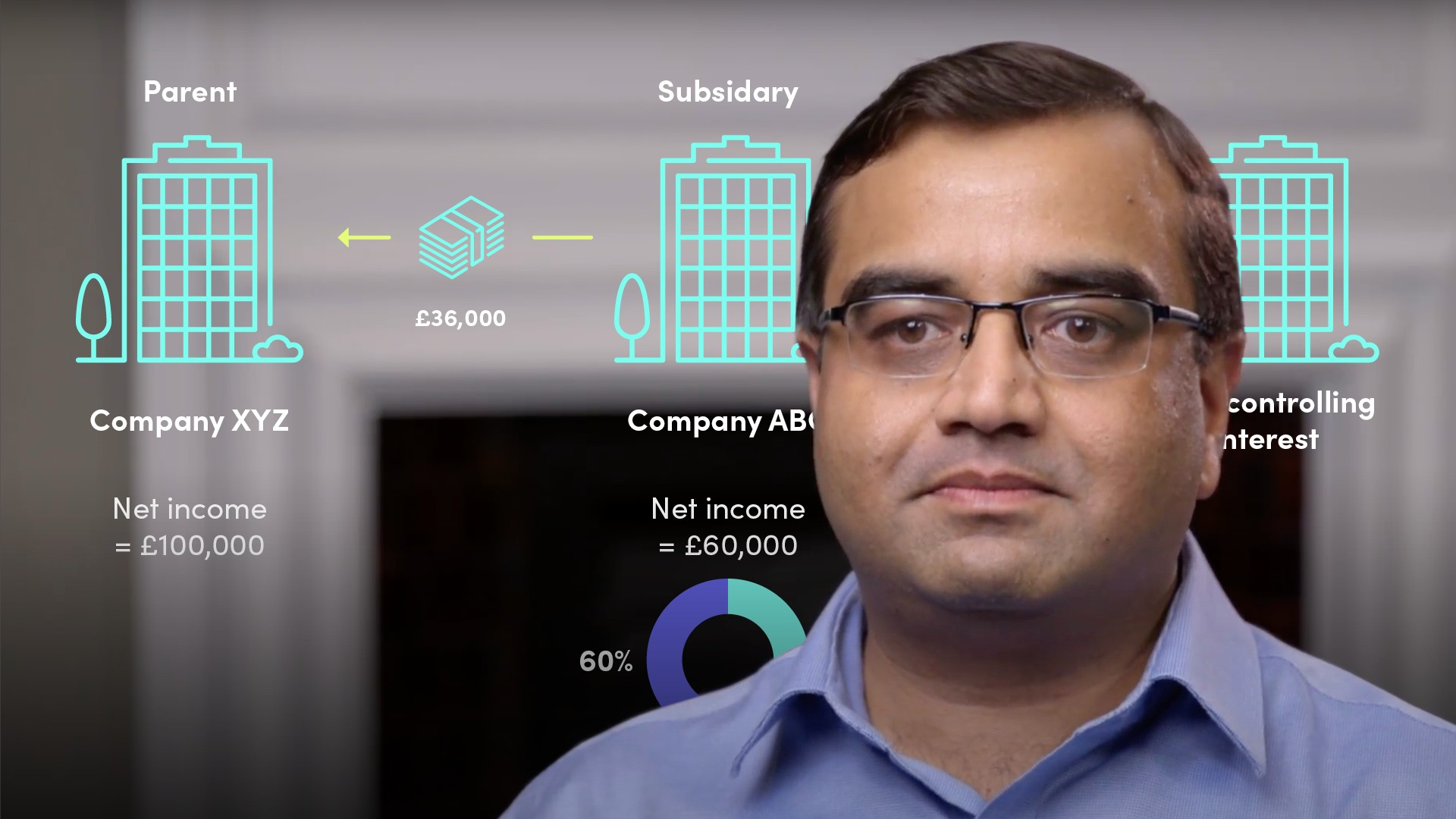04:16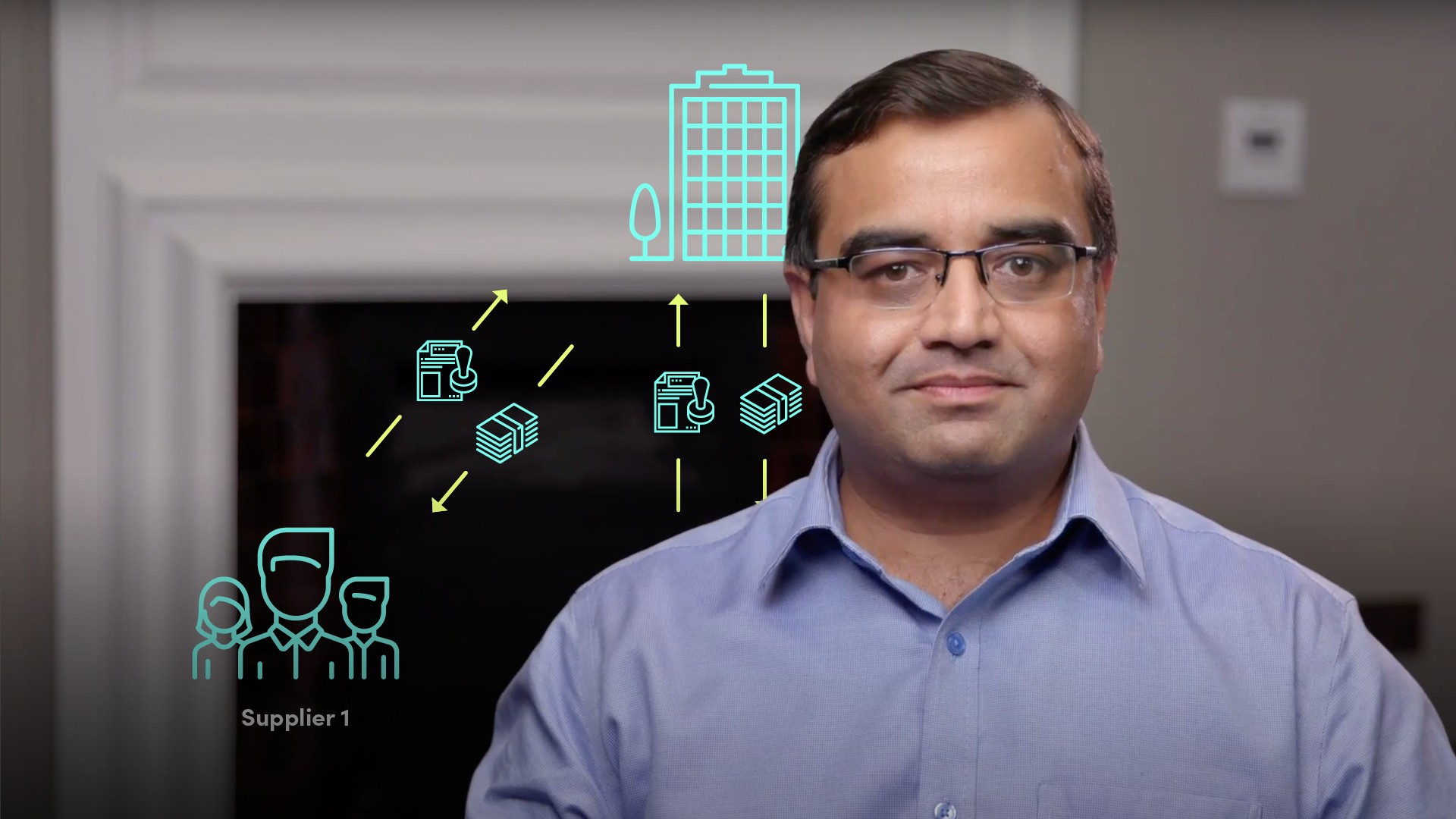08:42

## Related videos

### Accredited by